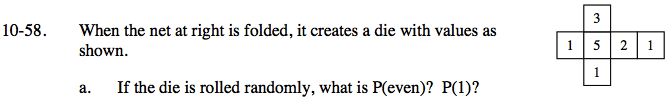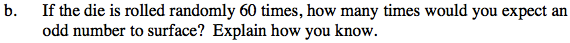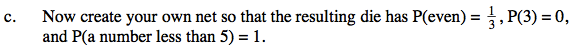### Home > GC > Chapter 10 > Lesson 10.2.1 > Problem10-58

10-58.Review the Math Notes box in Lesson 10.2.1.

$P(\text{even})=\frac{\text{number of sides on die with even numbers}}{\text{number of sides on die}}$Find the number of expected instances by multiplying the probability of the event by the number of attempts.

$P(\text{odd})=\frac{5}{6}$

$\frac{5}{6} \cdot 60= 50$One-third of the die faces should show even numbers.